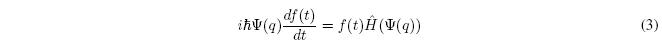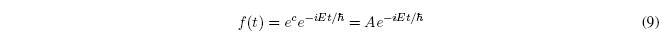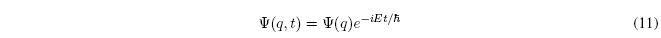When the Hamiltonian is independent of time, the state function can be written as a product of a function of time and a function of position.Substituting into the time-dependent Schrödinger equation:Since $\Psi$ does not depend on t and $\hat{H}$ does not depend on either, we can take $\Psi (q)$ from the time derivative and $f(t)$ from the Hamiltonian.Dividing the terms of equation (3) by $f(t)\Psi (q)$ gives us:The member on the left depends only on t and the one on the right only on the coordinates q. Thus, equality is only possible if both terms are equal to a constant that we will call E.The first equation gives us the temporal evolution of the system (temporal Schrödinger equation). The second equation is the spatial or time-independent Schödinger equation.
We integrate the temporal Schrödinger equation:where C is the constant of integration. Taking antilogarithms, the function f(t) is obtained.The constant A can be included in the function $\Psi (q)$ as a multiplicative factor, thus, we can write the above equation as:Therefore, in those systems in which the Hamiltonian does not depend on t, the state function can be written:The states of a physical system that can be written in the above way are characterized by having a constant energy and are called stationary states. Stationary states are characterized by having a probability density independent of time.Therefore, for a system with $\hat{H}$ independent of t, the total energy is constant and the probability that the particle has its coordinates between $q$ and $q+dq$ is not it changes over time.